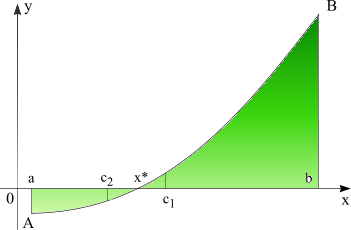# Bisection method

Numerical methods

Root bracketing methods

Root polishing methods

Bisection method is the simplest method of bracketing the roots of a function and requires an initial interval which is guaranteed to contain a root -- if a and b are the endpoints of the interval then f(a) must differ in sign from f(b). This ensures that the function crosses zero at least once in the interval. If a valid initial interval is used then these algorithm cannot fail, provided the function is well behaved.

On each iteration, the interval is bisected and the value of the function at the midpoint is calculated. The sign of this value is used to determine which half of the interval does not contain a root. That half is discarded to give a new, smaller interval containing the root. This method can be continued indefinitely until the interval is sufficiently small. At any time, the current estimate of the root is taken as the midpoint of the interval.

Bisection method has linear convergence. Linear convergence means that successive significant figures are won linearly with computational effort.

When an interval contains more than one root, the bisection method can find one of them. When an interval contains a singularity, the bisection method converges to that singularity.PROCEDURE Bisection(a,b,eps:Real; VAR xsol:Real);
{ Required condition: f(a)*f(b)<0 }
{ eps = accuracy of the root, e.g.: 0.000001 }
VAR
c:Real;
BEGIN
REPEAT
c:=(a+b)/2;
IF f(a)*f(c)<0 THEN b:=c
ELSE a:=c
UNTIL b-a<eps;
xsol:=c
END; {Bisection method - Pascal code}

Numerical methods | Bisection method | Regula Falsi method | Brent method | Newton method | Secant method

See how bisection method works, solve equations free and take the results with you.

DeadLine OnLine - free equation solver. Copyright 2003-2007 Ionut Alex. Chitu. | Contact | Sitemap